Mathematical game for addition, subtraction, multiplication tables and division.

APP Information

 Download Version 1.2 (3) Apk Size 8.28 MB App Developer Vasundhara Game Studios Malware Check TRUSTED Install on Android 4.1.x and up App Package com.maths.games.add.subtract.multiply.divide.apk MD5 3b49db09a5d4afbac141a033d8e598fc Rate 5### App Description

``` for everyone from kids to adults. Mathematical calculations to play and practice with simple addition subtraction multiplication and division. The mathe game division calculator is division app for kids as the maths division app as the math division game also call math divide app as math divide game math division app as math divition at the matematica. This is best math game brain maths as excercise math free maths games very hard math games for kids math brain math game apps. The math game for adults as the addition games as the math test plusminus as simple mathematics apps.

# Subtraction games subtracting numbers to solve the equations.

# Multiplication games multiplication tables learning and duel play mode.

# Division games practice and learn division tables.

# Maths times tables and hard games on maths.

Math Games - Maths Tricks in category has different play modes to improve math skills play learn quiz practice duel. Free math games can be educational learning for kids or brain training app for adults. This is maths solutions for any question and maths tricks is maths questions solving app as well as maths app as maths quiz. The maths games at the maths quiz games as the maths question solver app. The maths questions with answers as mathematics apps as mathematics books free at mathematics formula as well mathematics games mathematics games at the maths tables book free as the maths tables learn easy 1 to 30 as the maths tables games. The math games for adults offline as math master exercises. The mathematics addition math game as the multiplication and division games for 4th graders as the math practice set in hindi as the math practice set in hindi offline. In this app simple maths tricks in the simple maths for kids as the simple maths arithmetic tricks as the simple maths game arithmatic app or math apps math for kids. The simple addition for kids as the simple addition subtraction or simple multiplication or ganit sikhne ka tarika also ganit sikhne wala app at the ganit sikhne ke liye as math games for free subracting apps as simple mathematics games for kids all maths formula app as how to learn tables easily mathematics.

Math Games - Maths Tricks is maths practice game to train your brain & is designed for all ages including kids girls and boys adults including parents and grandparents. Smallest in size maths apps on google play easiest multiplication and division games with addition and subtraction games all in one app and maths tricks books free. The mathematics apps learn mathematics add subtract multiply & divide this is kides game and kides math as the math table math year 1. This is math year 1 as the math in hindi with solution at the brain games also mathmatics app in hindi at the mathmatics solution app for brain puzzle games for children as subtraction games for kids in this subtraction games 1st grade as the subtracting and adding at addition and subtraction games for kids as the hard math games for adults as hard mathematics game and mathematics hard games and math puzzle games as math practice app.

This addition subtraction multiplication and division games as addition subtraction and multiplication addition subtraction multiplication division. In this app addition subtraction app as the two digit addition and subtraction. This is easy math games for kids free easy maths learning app at the easy maths games easy maths quiz at the easy maths tricks. This is math answer app with solution multiplication table learning for kids at the multiplication table kids math as divides game.  ```

base.apk

### Apk scan results

```
Apk Scaned By TotalVirus Antivirus,com.maths.games.add.subtract.multiply.divide.apk Was Pure And Safe.

Scan Stats:harmless:0|type-unsupported:11|suspicious:0|confirmed-timeout:0|timeout:0|failure:0|malicious:0|undetected:61|
Name:base.apk
SHA-1:85deb4441a53bc30cd1a7b49ec6cb85926fd928c
SHA-256:53328e94ede036eaaa8fc5cea5ddab2c0d2f6cd38e88e69b0dc8f2697147fe58
SSDEEP:196608:Hxg6fLtST3txlrE+PNqV16f6q5HeNOW9TO88D82Ip:RZDK5hqV16yqReNOkh8D89
File type:Android
Magic:Zip archive data
File size:8687315
Uncompressed Size:15983618
Contained Files	:947
Contained Files By Type:xml:254,dex:2,MF:1,RSA:1,jpg:2,ttf:13,mp3:3,sql:1,wav:2,txt:125,SF:1,png:521,
```

### More Android Apps to Consider

1.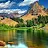Mominul Karim

This game want permission of storage where other games don't need.

2.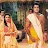Pratibha Gupta

This is good app. It is useable and very interesting. I always play this game with my full interest. I prefer this game to everyone. But, this game should also be online game app. I like playing this game. If I add more, I will say that this is a useful game and easy way to learn dodging tables and division. This is a free time playing game.The game is easy but somewhere it is tricky also. A good study at home by mobile phone. Play it after your tuitions or before your tuitions.

3.Sam Towers

I just want to be clear there is a major problem with the subtraction. From level 1 the sums are answers that go into the negative. My child is in year 2 and when they are tought subtraction they don't learn about subtracting into the negative. Subtraction for any child is hard enough first up and then getting those sort of sums just confuses the situation. Maybe in higher levels but not from level 1. It confuses the child and turns them off the learning

4.Akanksha T Shukla

I am Aashvi Shukla I am four and a half years old I LOVE this App because i love math this. Multiplication(*) i know multiplying small numbers. Division(/) i dont know Addition(+) i know adding small numbers like 4+3 5+5 Subtraction(-) i know subtracting small numbers like 4-4 5-5 I love the number 7 Since i was 2 and a half i loved math!!!!!!!!!!!!!!!!!!!!!!! I learned math so i love math I love math My mom and dad and teachers taught me i like this game name called "Math Games - Maths Tricks"

5.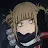•SavWølf•

i have not tryed it yet but i see feeeback saying " why is there a time? i dont wanna be timed!" if you're a kid it does that coz what if you have a math test and you are being timed to do it this games make you go faster so you can go faster at your test

6.Couch potato :3

I think this app is great because the picture is very good it has math questions that that aren't too challenging but they are not too easy. It's just over all a great game 100% should download it!

7.David Gaudet

Not bad pretty good game for math thought it was pretty good for time and you knowgot everything is correct. It was pretty good learning experienceyou guys want to go all the way to make this happen all the way around the world probably good idea

8.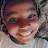Gaming with Tania Chings

you can get so smart at this my daughter needs to feel nauseous or small or thank you god for giving us this up at least it makes our children so smart I have a little boy but doesn't know anything while I told him to download that app and he did he got smart and small and small and small just same as my daughter very smart than him there even know how to read them before

9.Traji coates

In my opinion i think that when u do division don't put a line instead of ÷ it confuse me I didn't know it was division but I pressed all

10.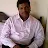Shanmugam

Great.It improved my daughter a lot in Maths. Very helpful Please download this app if u are weak in Maths.🤩😉 But You should increase the difficultly of the levels. Thank you .😀